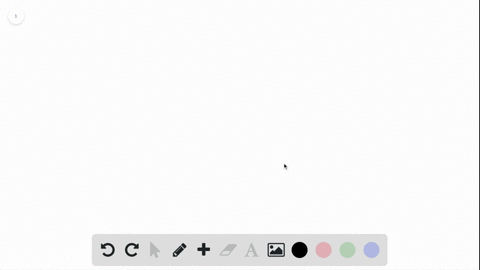Enroll in one of our FREE online STEM bootcamps. Join today and start acing your classes!View Bootcamps### A team's best hitter has a lifetime batting avera…

00:35Problem 32

# What is the ratio of the measure of an interior angle to the measure of an exterior angle in a regular hexagon? A regular decagon? A regular $n$ -gon?

## Discussion

You must be signed in to discuss.

## Video Transcript

Okay, So in the next question, which is questioned 32 this is what is the ratio of measure? Oven interior angle to measure oven exterior angle in a regular hexagon. Now, if you're not sure Hey, a hexagon on a hexagon has six sides. Six eyes. It doesn't say regular hex of, um Um, yes, it does. Regular hexagon means all the sides are equal. That's very key. A regular hexagon has All the sides are equal. So what? It's actually asking us here. It's actually asking us for the ratio of the measure. Often interior angle to the measure of an exterior angle in a regular hexagon. Okay, so is asking for the ratio of this angle to that angle. Now, remember, these are called, um, consecutive angles there, same side. They're the angle which lie in a straight line, the consecutive angles which fly in a straight line. So they add to 1 80 on 280. Okay, so in ah, hacks a gone. Okay. In order to get each angle in general, as long as it's regular regular, you take 3 60 divide by the number of sides, and that gives you the angle inside. So if this is 60 this will be 1 20 because I have to add to 1 80 So the interior to the exterior would be 60 to 1 20 which would be one, 22 Now there's multiple parts of this question. Now it says a regular duck A gum. You may be saying, Oh, I don't know how to draw that. That's okay. A duck a gone has 10 sides, and again it's regular. So just pretend you have the interior angle on the exterior angle. Okay, so you do 3 60 divided by 10. This gives you the measure of an interior angle. So 36 isn't interior angle. In order to find the exterior angle, we're gonna do 1 80 minus 36 which we would get to be 1 44 So the ratio would be 36 to 1 44 which should be one. Just for that would be for a regular deca God. Now it says a regular end gone a regular end. God. Okay, so this is a little trickier for a regular and gone and gone just means any number of sides remember to find this angle inside. We d'oh! 360 divided by Ed. Okay, that's gonna be this angle in here. Okay? Now, this is when it becomes a little trickier, because the outside angle here is gonna be 1 80 minus 3 60 over at. Okay, Now what you want to do? And again, this would be kind of like a bonus question I would assume for a lot of teachers, okay, is you want to get a common denominator, You'd want to get a common denominator of end so you would get 1 80 minus 3 60 over and And that would be the outside angle. And if you added this to this, you would see it would come out to 1 80 But now we actually have to do the ratio. So 360 over and over 1 80 minus 3 60 over end. As long as you dividing, remember, you're changing to multiplication and using the reciprocal these ends, we're gonna go away. Okay? So what, you're gonna have laughed and try it out for yourself? It works. What you're gonna have left is 360 3 60 over. Okay. To get if you need to go back to the other 11 80 and minus 3 61 80 and minus 3 60 And at that point, you want this ratio, you can factor this out 1 80 and minus two. And you'd be left with 360 over 1 80 and minus two. So your ratio be too over and minus two. This one is tricky.